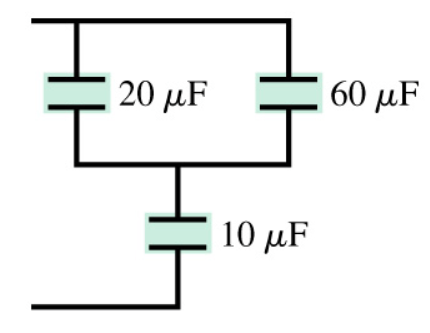# Problem: What is the equivalent capacitance of the three capacitors in the figure?

###### FREE Expert Solution

Equivalent capacitance for series capacitors:

$\overline{)\frac{\mathbf{1}}{{\mathbf{C}}_{\mathbf{e}\mathbf{q}}}{\mathbf{=}}\frac{\mathbf{1}}{{\mathbf{C}}_{\mathbf{1}}}{\mathbf{+}}\frac{\mathbf{1}}{{\mathbf{C}}_{\mathbf{2}}}}$

Equivalent capacitance for parallel capacitors:

$\overline{){{\mathbf{C}}}_{\mathbf{e}\mathbf{q}}{\mathbf{=}}{{\mathbf{C}}}_{{\mathbf{1}}}{\mathbf{+}}{{\mathbf{C}}}_{{\mathbf{2}}}}$

The 20 μF and 60 μF are in parallel:

C20,60 = C20 + C60 = 20 + 60 = 80 μF###### Problem Details

What is the equivalent capacitance of the three capacitors in the figure?